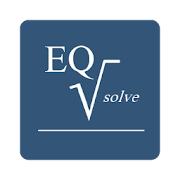# EQSolveEveryone
17
EQSolve is designed for those times when you need an answer to a well-known equation quickly. Instead of having to remember the equation you're working on, rearranging the terms and then calculating each part separately with a normal calculator, EQSolve does it for you. Simply select the equation you want to use, choose the variable to calculate, fill in the known variables and calculate the answer. No more pen and paper!

EQSolve includes 15 equations:
Boyle's Law
Charles' Law
Coulomb's Law
Einstein's Equation of General Relativity
Hooke's Law
Hubble's Law
Ideal Gas Law
Newton's Law of Gravitation
Newton's Second Law
Ohm's Law
Pythagoras' Theorem
Snell's Law
Trigonometry: cosine
Trigonometry: sine
Trigonometry: tan
Collapse

## Reviews

Review policy and info
4.4
17 total
5
4
3
2
1

## What's New

Changes in v1.1:

* bug related to incorrect calculate button positioning in some devices is fixed
* bug related to units being placed slightly off-screen in some devices is fixed
* support for small screens (eg HTC Wildfire) introduced
* small changes to look and feel
Collapse

Updated
March 4, 2017
Size
64k
Installs
5,000+
Current Version
1.2
Requires Android
1.6 and up
Content Rating
Everyone
Permissions
Offered By
Particle Drift